Question

Hydraulics and Pneumatics

Capside area of each actuator = Acap = 2A

Rodeside area of each actuator = Arod = A%3D

The viscous friction force in each actuator:

Actuator 2: F2 = b2 V2

Actuator 1: F, = b, v,

where v,and v, are the velocities of the respective actuators.

a) The figure below shows the two actuators connected in parallel driven by variable flow pump.a

Determine the relationship between pump flow Q and pump pressure p (interms of b,, b, and A)

• Sketch p vs Q

Assuming b, > b2 which actuator moves faster?

1In this problem, we consider two actuators connected in parallel and in series.

b) Figure below shows the two actuators connected in series also driven by a variable flow pump.

Find actuator speeds v, and v, as functions of pump flow Q

Find relationship between p and Q and sketch p vs Q.Verified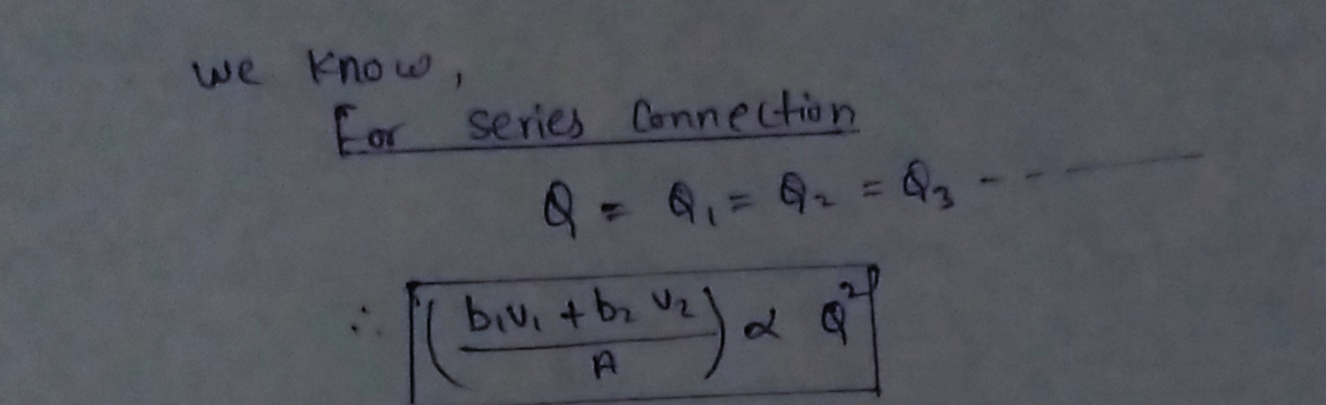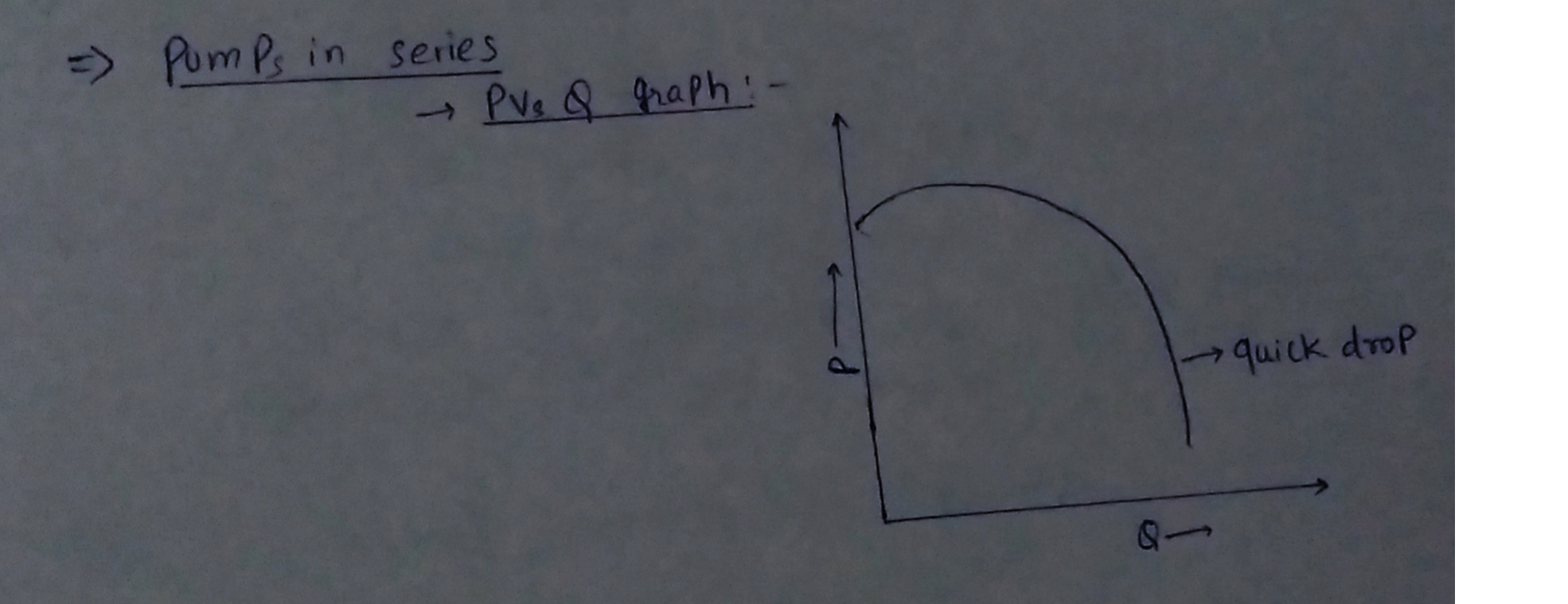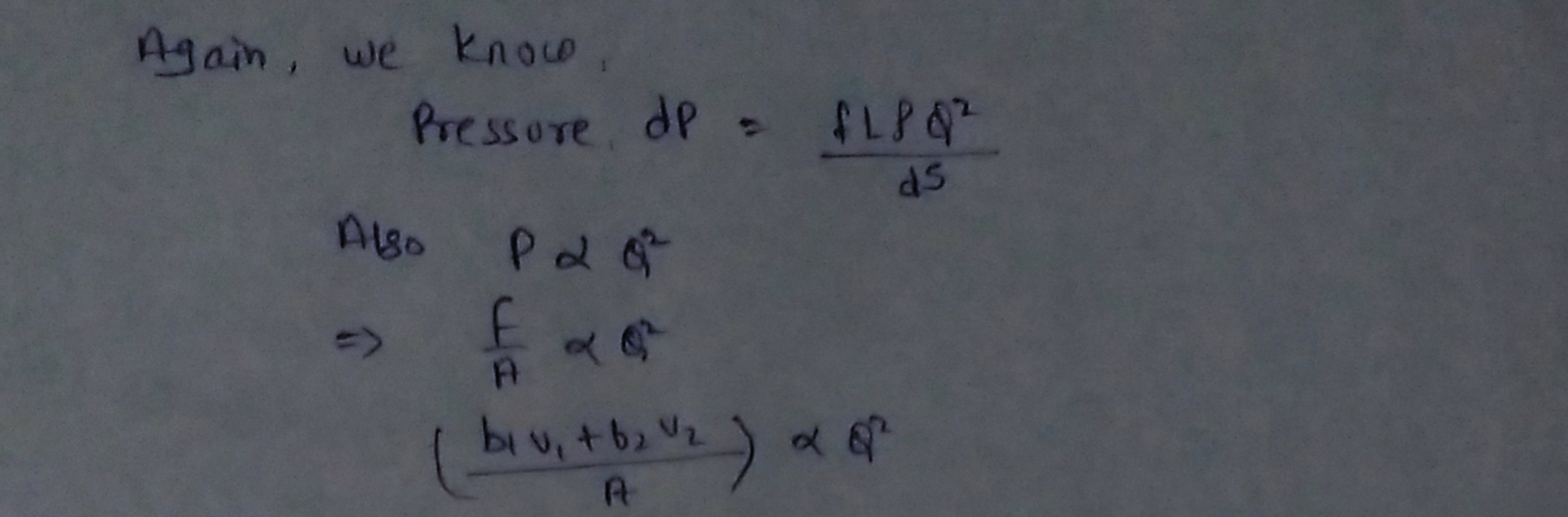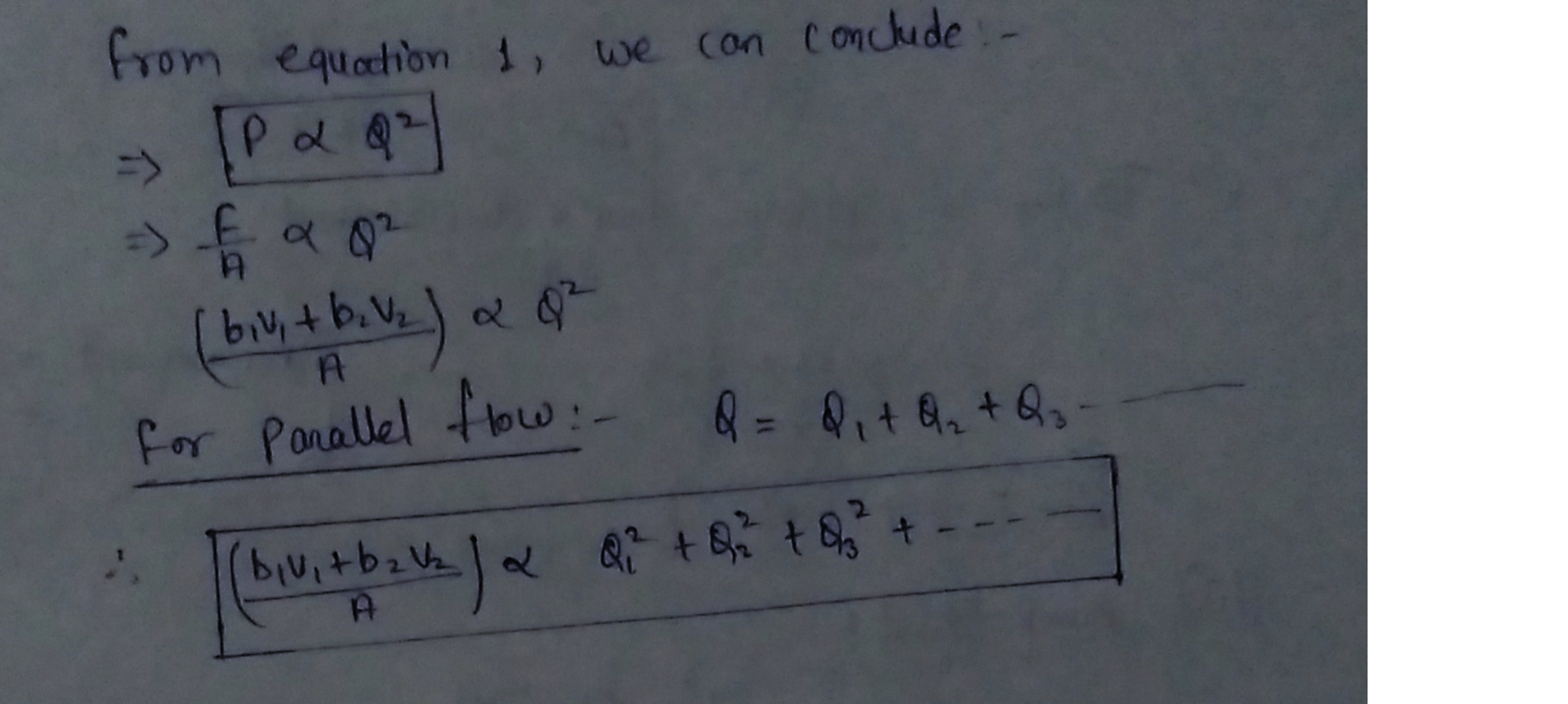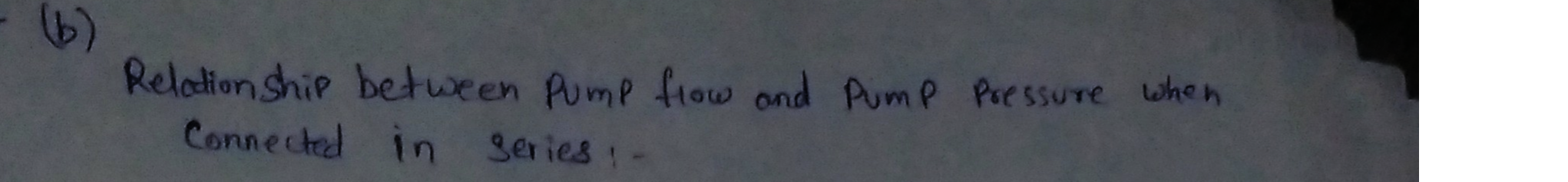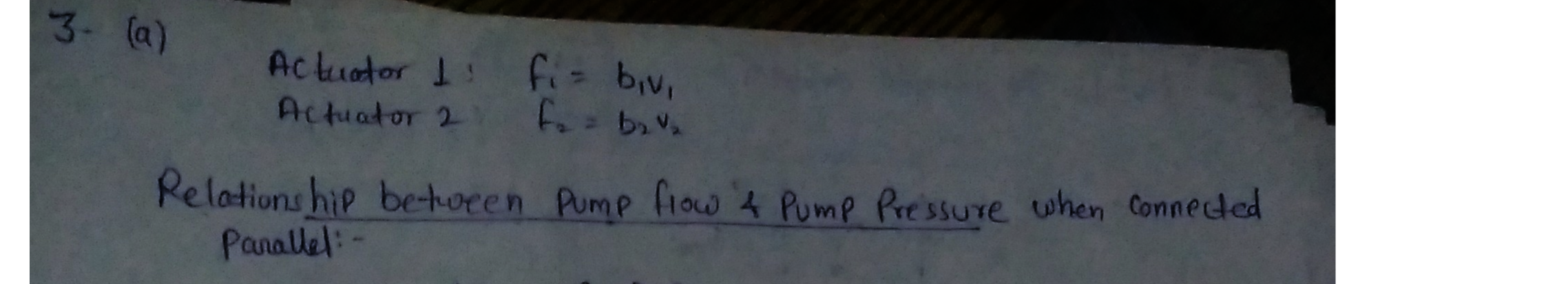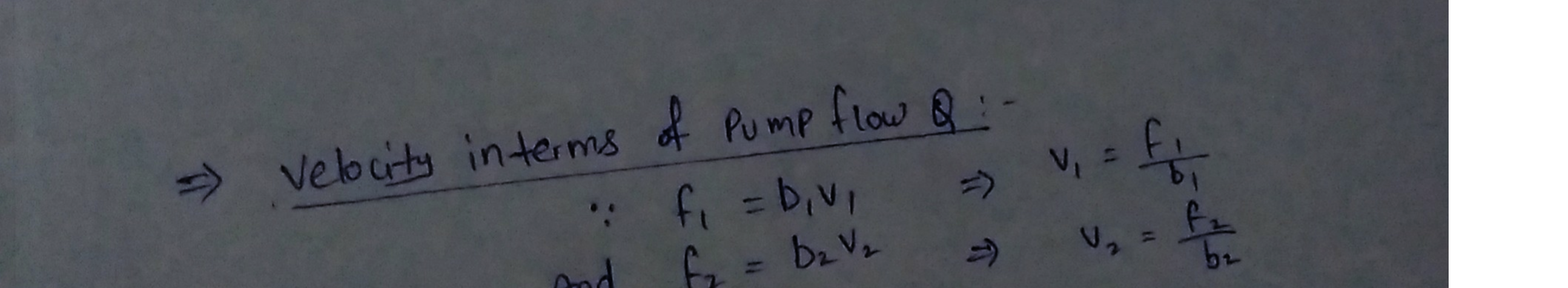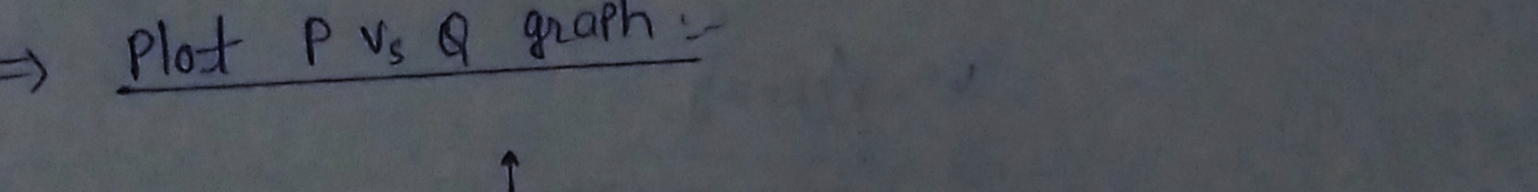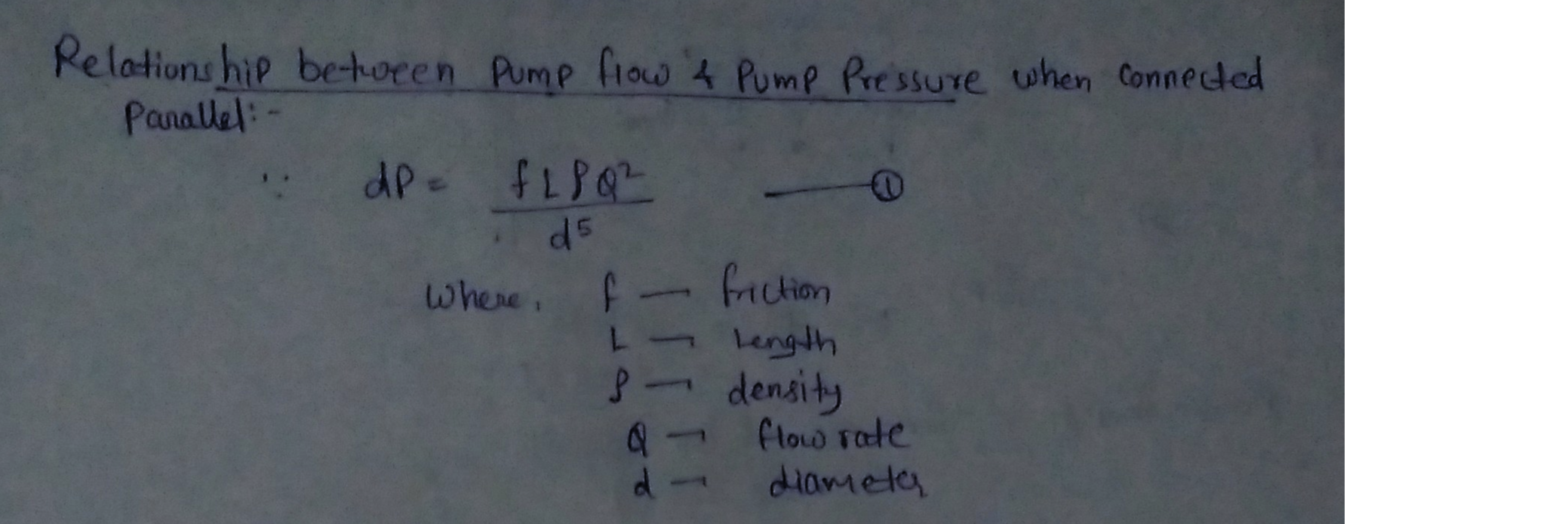### Question 40658Hydraulics and Pneumatics

Capside area of each actuator = Acap = 2A
Rodeside area of each actuator = Arod = A%3D
Actuator 2: F2 = b2 V2
The viscous friction force in each actuator:
Actuator 1: F, = b, v,
where v,and v, are the velocities of the respective actuators.
a) The figure below shows the two actuators connected in parallel driven by variable flow pump.a
Determine the relationship between pump flow Q and pump pressure p (interms of b,, b, and A)
• Sketch p vs Q
Assuming b, > b2 which actuator moves faster?
1In this problem, we consider two actuators connected in parallel and in series.
b) Figure below shows the two actuators connected in series also driven by a variable flow pump.
Find actuator speeds v, and v, as functions of pump flow Q
Find relationship between p and Q and sketch p vs Q.

### Question 40657Hydraulics and Pneumatics

2. [25 pts] Consider the electro-hydraulic actuator system below in which an electric motor driven pump is used to control a linear actuator. The pump is assumed to be ideal.
Weight of the mass: W = Mg = 1e4 N
Actuator piston area: Ap = 0.001 m?
a) [10pts] Ignoring the needle valve, find i) pressure drop Ap[Pa], ii) ideal pump torque T ideal [N], and iii) ideal pump speed wheel [rad/sec] in order to raise the mass at a speed of v=0.1m/s.
Pump displacement: D = 20cc/rev
b) [15 pts] Consider now that the needle valve is in place representing leakage. It is known that the valve has these characteristics:
\mathrm{Q}_{\text {leak }}=5 \mathrm{cc} / \mathrm{s} \text { when pressure drop is } \Delta p=5 \mathrm{MPa}
Find i) the orifice flow Qleak, ii) the new pump torque T [Nm] and iii) new pump speed w[rad/sec] in order to raise the mass at a speed of v=0.1m/s.
Determine also iv) the power loss at the needle valve.

### Question 35406Hydraulics and Pneumatics

1. State all assumptions and determine friction loss in a concrete pipe (L = 3000 ft, D = 1 ft) that carries 12 cfs of water at 140°F.
a) Use Darcy-Weisbach equation
b) Use Hazen-Williams equation
d) Briefly explain why selection of a design value for pipe roughness is appropriate for new pipe
c) Briefly explain any difference between energy loss calculated by both methods

### Question 35405Hydraulics and Pneumatics

2. A storage tank discharges water @ 20°C through a galvanized iron pipe (L = 25 m, D = 150 mm).Ignore minor loss and assume pipe is horizontal.
a) Calculate required depth of water above pipe outlet to deliver 0.10 m³/sec to customers
b) Estimate pressure (kPa) in middle of discharge pipe.

### Question 34711Hydraulics and Pneumatics

A circular hydrostatic bearing with an outside diameter of 150 [mm], recess diameter of 60 [ mm] and rotating at 500 [rpm] supports a load of 50 [kN]. The bearing is lubricated by a mineral oil of density p = 900 [kg/m] delivered to the bearing by a constant flow rate delivery pump operating at 50×10* [m°s"].The operating temperature of the bearing is 30°C.
calculate the recess pressure and
select an SAE oil such that the film thickness will never be less than 10 [um]. (Ans.P, = 6.173 [MPa], SAE20)

### Question 34710Hydraulics and Pneumatics

For the bearing specified in problem 6.11 calculate the percentage in load increase that would cause a 20 percent decrease in film thickness

### Question 34709Hydraulics and Pneumatics

A pump capable of maintaining 20 [MPa] of pressure is supplying oil of viscosity 80 [cP] to a circular hydrostatic thrust bearing of an outside diameter of 0.1 [m] at a rate of 0.4x10* [m/s], generating a steady film thickness of 30 [um]. The bearing supports a weight of 60 [kN] and rotates at 1,000 [rpm]. Calculate a suitable recess radius and pumping power. (Ans. R, = 0.0285 [m], H= 50.89 [W])

### Question 34708Hydraulics and Pneumatics

A circular hydrostatic bearing operates at the speed of 200 [rps], has a minimum film thickness of 50 [um]and supports a load of 50 [kN]. The bearing's outside radius is three times larger than the recess radius.A lubricant is delivered through the capillary length of 1 = 80 [mm] and diameter d = 0.7 [mm]. The pump supply pressure is 3 [MPa] and the lubricant's viscosity is 8.5 [cP]. Calculate bearing dimensions, friction coefficient and power loss. (Ans. R, = 0.051 [m], R = 0.154 [m], µ = 0.0005 and H = 27.32 [W])

### Question 34707Hydraulics and Pneumatics

For the bearing specified in problem 6.8 calculate the capillary dimensions necessary to maintain the minimum film thickness. The pump supply pressure is 3 [MPa]. (Assume 1/d = 100, where I' is the length while 'd' is the diameter of the capillary). (Ans. d = 0.8 [mm], 1 = 80 [mm])

### Question 34706Hydraulics and Pneumatics

A hydrostatic circular pad bearing operating under a minimum oil film thickness of 50 [um] supports vertical load of 50 [kN] at a shaft speed of 2,000 [rpm]. The lubricant's viscosity at the operating temperature is 8.5 [cP], the recess oil pressure is 2 [MPa] and the external pressure outside the bearing isP. = 0. Assuming that D/D. = 3, calculate:
the bearing dimensions,
the rate of oil flow through the bearing,
the power losses 'H' due to viscous friction in the bearing and
the coefficient of friction (assume that the friction force is acting at the mean radius of the bearing area). (Ans. D, = 0.095 [m], D = 0.282 [m], Q = 1.402x10° [m°/s], H = 3.69 [kW], µ = 0.004)

### Submit query